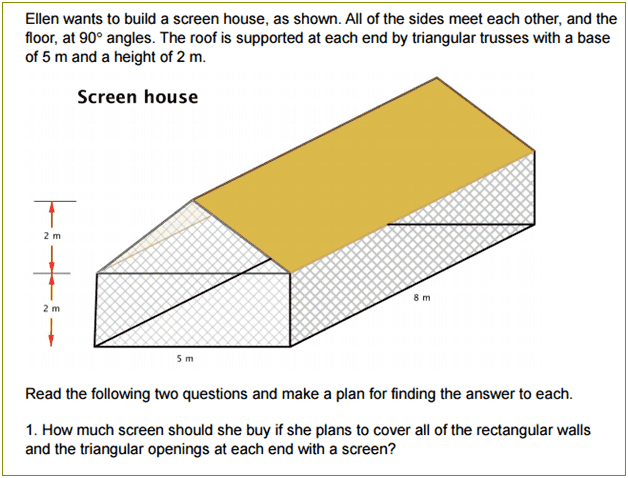# Finding area of shapes.

## Homework Statement2. Homework Equations

A = l x w
A = b x w / 2

## The Attempt at a Solution

I calculated all the areas for the triangles and rectangles. So for the first rectangle (the long one) I did (L)(w) = A. Basically 8 x 2 = 16m2 (this is the same area for the other long rectangle). Now to find the smaller rectangle ill use the same formula A= (L)(W), So 5 x 2 = 10. The area for the smaller rectangles is 10m2. Now all I have left is to find the area of the triangles. I’ll use the formula A = H x B/2. So 2 x 5 = 10, 10/2 = 5m2. So the areas for both triangles are 5m2.

So now I am going to add all the areas I got so that I can determine how much screen I need to cover all the walls.

16 + 16 + 10 + 10 + 5 + 5 (Note: I did 16+16 since both rectangles are parallel same with 10+10 and 5+5)

= 62m2

Ellen will need about 62m2 of screen to cover all of the rectangular walls and the triangular openings at each end with screen.

I don't know if the way i did is what they were asking for. Any help will do thx :)